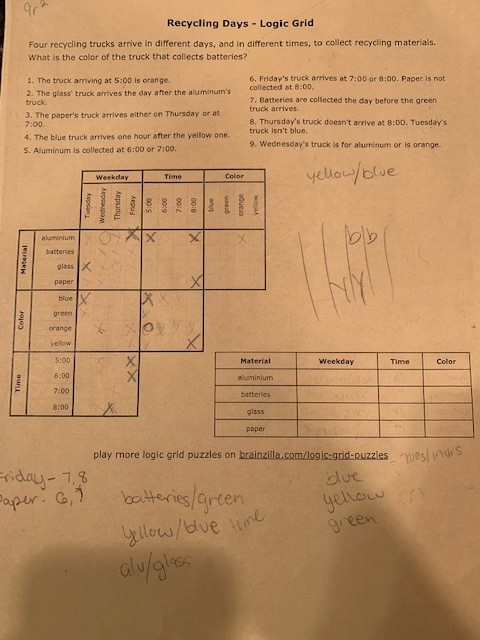## Posts

Showing posts from April, 2021

### Employee Importance: Cache + DFSHere is a problem (marked as easy) on LC:  Employee Importance - LeetCode 690. Employee Importance Easy 989 896 Add to List Share You are given a data structure of employee information, which includes the employee's  unique id , their  importance value  and their  direct  subordinates' id. For example, employee 1 is the leader of employee 2, and employee 2 is the leader of employee 3. They have importance value 15, 10 and 5, respectively. Then employee 1 has a data structure like [1, 15, ], and employee 2 has [2, 10, ], and employee 3 has [3, 5, []]. Note that although employee 3 is also a subordinate of employee 1, the relationship is  not direct . Now given the employee information of a company, and an employee id, you need to return the total importance value of this employee and all their subordinates. Example 1: Input: [[1, 5, [2, 3]], [2, 3, []], [3, 3, []]], 1 Output: 11 Explanation: Employee 1 has importance value 5, and he has two direct subordinates: employee

### Logic Grid using BacktrackingMy daughter had an assignment to solve a logic grid - recycling days. The puzzle is in the image below. She needs to finish it the old-fashioned-raw-logic way (which she is doing), but I decided to take a brute-force approach. This is a typical backtracking problem. The approach should be as follows: 1/ Figure out how to model the problem. I decided to model yet using an array of "days" where each day will have a truck, a material and a time 2/ Start by creating the validation function. This is the boring and detailed part that you can't mess up. Just a matter of reading the problem definition and implementing each one of the rules 3/ Then comes the backtracking. DFS using hash tables to keep track of which elements have already been used Let it run for ~1sec. It outputs the result. I have not shared the results with my daughter... Result and code are below, cheers, ACC. using System; using System.Collections; namespace RecyclingDays { public class Days {

### Removing duplicates from a linked list (linear time and linear space)I'm sure there is a way to do this in linear time and constant space, but the solution below is linear on both time and space. Here is the problem:  Remove Duplicates From an Unsorted Linked List - LeetCode 1836. Remove Duplicates From an Unsorted Linked List Medium 16 1 Add to List Share Given the  head  of a linked list, find all the values that appear  more than once  in the list and delete the nodes that have any of those values. Return  the linked list after the deletions.   Example 1: Input: head = [1,2,3,2] Output: [1,3] Explanation: 2 appears twice in the linked list, so all 2's should be deleted. After deleting all 2's, we are left with [1,3]. Example 2: Input: head = [2,1,1,2] Output: [] Explanation: 2 and 1 both appear twice. All the elements should be deleted. Example 3: Input: head = [3,2,2,1,3,2,4] Output: [1,4] Explanation: 3 appears twice and 2 appears three times. After deleting all 3's and 2's, we are left with [1,4].   Constraints: The num

### The Circle EquationProblem is actually very simple - all you have to do is remember the equality (or inequality) for the circle in 2D. Here it is:  Queries on Number of Points Inside a Circle - LeetCode 1828. Queries on Number of Points Inside a Circle Medium 27 8 Add to List Share You are given an array  points  where  points[i] = [x i , y i ]  is the coordinates of the  i th  point on a 2D plane. Multiple points can have the  same  coordinates. You are also given an array  queries  where  queries[j] = [x j , y j , r j ]  describes a circle centered at  (x j , y j )  with a radius of  r j . For each query  queries[j] , compute the number of points  inside  the  j th  circle. Points  on the border  of the circle are considered  inside . Return  an array  answer , where  answer[j]  is the answer to the  j th  query .   Example 1: Input: points = [[1,3],[3,3],[5,3],[2,2]], queries = [[2,3,1],[4,3,1],[1,1,2]] Output: [3,2,2] Explanation: The points and circles are shown above. queries is the green circ

### On Divergent and Convergent SeriesIn a math post, I saw a question that was intriguing: At a first glance, the first series seems to grow much faster than the second one. But in fact, there is something quite interesting about these two series. The first one was a problem solved by Euler, the so-called the Basel Problem  Basel problem - Wikipedia . The series actually is convergent, and it converges to PI^2 / 6, also known as 1.644934066848226436472415166646... So series1 < 1.65. The second one, on the other hand, can be transformed into something that is well-known. What you can do is multiply both sides to 40, ending up with the following equation: The part in red of the equation is the famous Harmonic Series  Harmonic series (mathematics) - Wikipedia . Which, despite the very slow growth rate, it is a divergent series, meaning that it keeps growing  ad infinitum .  Hence, the answer to the question is that, eventually, Series2 >> Series1. But when exactly does Series2 become larger than Series1? That's